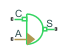# Rotational Hydro-Mechanical Converter

Interface between hydraulic and mechanical rotational domains

## Library

Hydraulic Elements

•## Description

The Rotational Hydro-Mechanical Converter block models an ideal transducer that converts hydraulic energy into mechanical energy, in the form of rotational motion of the converter shaft, and vice versa. Physically, the converter represents the main component of a single-acting rotary vane actuator. The compressibility option makes the converter account for dynamic variations of the fluid density.

Using this block as a basic element, you can build a large variety of rotary actuators by adding application-specific effects, such as leakage, friction, hard stops, and so on.

The converter is simulated according to the following equations:

`$q=\frac{d\left(\frac{\rho }{{\rho }_{l}^{0}}V\right)}{dt}=\frac{d\left(\frac{\rho }{{\rho }_{l}^{0}}\right)}{dt}V+\frac{\rho }{{\rho }_{l}^{0}}\cdot \epsilon \cdot \left({\omega }_{S}-{\omega }_{C}\right)\cdot D$`
`$T=\epsilon \cdot p\cdot D$`

where

 q Flow rate to the converter chamber D Converter displacement, or fluid volume needed to rotate the shaft per angle unit ωS Converter shaft angular velocity ωC Converter case angular velocity T Torque on the shaft p Gauge pressure of fluid in the converter chamber V Piston volume α Relative amount of trapped air ρl0 Fluid density at atmospheric conditions ρg0 Gas density at atmospheric conditions p0 Atmospheric pressure γ Specific heat ratio βl Bulk modulus at atmospheric conditions and no gas ε Mechanical orientation of the converter (`1` if increase in fluid pressure causes positive rotation of R relative to C, `-1` if increase in fluid pressure causes negative rotation of R relative to C)

The piston volume is computed according to

`$\begin{array}{l}V={V}_{dead}+D\cdot \left({\theta }_{0}+\theta \right)\\ \frac{d\theta }{dt}=\epsilon \cdot \left({\omega }_{S}-{\omega }_{C}\right)\end{array}$`

where

 Vdead Chamber dead volume θ0 Shaft initial angle θ Shaft rotation from initial position

Port A is a hydraulic conserving port associated with the converter inlet. Ports S and C are mechanical rotational conserving ports associated with the shaft and the case of the converter, respectively. Pressure at port A generates torque in the direction specified by the Converter orientation parameter.

The block dialog box does not have a Source code link. To view the underlying component source, open the following files in the MATLAB® editor:

• For incompressible converter implementation — `rotational_converter_incompressible.ssc`

• For compressible converter implementation — `rotational_converter_compressible.ssc`

## Basic Assumptions and Limitations

The block simulates an ideal converter, with an option to account for fluid compressibility. Other effects, such as hard stops, inertia, or leakage, are modeled outside of the converter.

## Parameters

Displacement

Effective converter displacement. The default value is `1.2e-4` m^3/rad.

Converter orientation

Specifies converter orientation with respect to the globally assigned positive direction. The converter can be installed in two different ways, depending upon whether it generates torque in the positive or in the negative direction when pressure is applied at its inlet:

• ```Pressure at A causes positive displacement of R relative to C``` — Increase in the fluid pressure results in a positive rotation of port R relative to port C. This is the default.

• ```Pressure at A causes negative displacement of R relative to C``` — Increase in the fluid pressure results in a negative rotation of port R relative to port C.

Compressibility

Specifies whether fluid density is taken as constant or varying with pressure. The default value is `Off`, in which case the block models an ideal transducer. If you select `On`, the block dialog box displays additional parameters that let you model dynamic variations of the fluid density without adding any extra blocks.

Shaft rotation

Select method to determine rotation of port R relative to port C:

• `Calculate from velocity of port R relative to port C` — Calculate rotation from relative port velocities, based on the block equations. This is the default method.

• `Provide input signal from Multibody joint` — Enable the input physical signal port q to pass the rotation information from a Multibody joint. Use this method only when you connect the converter to a Multibody joint by using a Rotational Multibody Interface block. For more information, see How to Pass Position Information.

This parameter is enabled when Compressibility is `On`.

Initial shaft angle

Initial offset of the piston from the cylinder cap. This parameter is enabled when Compressibility is `On` and Shaft rotation is ```Calculate from velocity of port R relative to port C```. The default value is `0`.

Volume of fluid in the chamber at zero piston position. This parameter is enabled when Compressibility is `On`. The default value is `1e-4` m^3.

Specific heat ratio

Gas-specific heat ratio. This parameter is enabled when Compressibility is `On`. The default value is `1.4`.

Initial pressure

Initial pressure in the chamber. This parameter specifies the initial condition for use in computing the block's initial state at the beginning of a simulation run. It is enabled when Compressibility is `On`. The default value is `0`.

## Ports

The block has the following ports:

`A`

Hydraulic conserving port associated with the converter inlet.

`S`

Mechanical rotational conserving port associated with the shaft of the converter.

`C`

Mechanical rotational conserving port associated with the case of the converter.

`P`

Physical signal input port that passes the position information from a Simscape™ Multibody™ joint. Connect this port to the position sensing port q of the joint. For more information, see Connecting Simscape Networks to Simscape Multibody Joints. To enable this port, set the Interface rotation parameter to ```Provide input signal from Multibody joint```.

## Version History

Introduced in R2007a